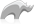##### [ loop ]

Method

[Loop] executes the provided body of code a specified number of times. A single parameter specifies the number of times the body should loop. The [Loop_Count] method can be used within the body of code to return the value of the current loop from the start value up to the end value.

Optional -From and -To parameters allow the loop to loop between any two values. [Loop(-From=11, -To=20)] ... [/Loop] will loop 10 times and [Loop_Count] will report the values 11, 12, 13, ..., 20.

An optional -By parameter allows the increment of the loop to be changed. [Loop(-To=10, -By=2)] ... [/Loop] will loop 4 times and [Loop_Count] will report the values 1, 3, 5, 7, 9.

The -By parameter can be used with the -From and -To parameters to loop down. [Loop(-From=10, -To=1, -By=-1)] ... [/Loop] will loop 10 times and [Loop_Count] will report the values 10, 9, 8, ..., 1.

[Loop] may use either signature form, with named parameters and integers or only integers corresponding to the position of the named parameters.

• Syntax
``````loop(-to=Integer Value, -from=Integer Value, -by=Integer Value) ... /loop
loop(Integer Value, Integer Value, Integer Value) ... /loop``````
• Beginner
• Intermediate

### To repeat a body of code a specified number of times:

Use the [Loop] method.

Code

``````loop(-from=11, -to=20)
loop_count + ' '
/loop``````

Result

`11 12 13 14 15 16 17 18 19 20 `

### To repeat a body of code a specified number of times:

Use the [Loop] method.

Code

``````loop(-to=10, -by=2)
loop_count + ' '
/loop``````

Result

`1 3 5 7 9 `

### To repeat a body of code a specified number of times:

Use the [Loop] method.

Code

``````loop(-from=10, -to=1, -by=-1)
loop_count + ' '
/loop``````

Result

`10 9 8 7 6 5 4 3 2 1 `

Loop is an excellent candidate for using capture blocks. Can be used with or without auto-collect (^...^ or caret).

Code

``````// How to output without auto-collect
var(my_total = 0)
loop(5) => {
\$my_total += loop_count
}
\$my_total

// How to output with auto-collect:
loop(5) => {^
'<br>This is loop '
loop_count
^}``````

Result

```15
This is loop 1
This is loop 2
This is loop 3
This is loop 4
This is loop 5```

To create a loop with minimal coding skip the named parameters and use capture block.

Note that [loop_count] in this case will reflect the increment steps and not how many times the loop has executed. If you need to know the number of loops you need to create your own counter.

Code

``````loop(30, 5, 5) => {^
'<br>'
loop_count
^}
<br>
var(my_counter = 0)
loop(30, 5, 5) => {^
\$my_counter += 1
'<br>'
\$my_counter
^}``````

Result

```5
10
15
20
25
30
1
2
3
4
5
6 ```Please note that periodically LassoSoft will go through the notes and may incorporate information from them into the documentation. Any submission here gives LassoSoft a non-exclusive license and will be made available in various formats to the Lasso community.